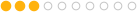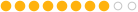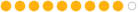### get all points from Wrangle (detail)

7122   7   2Member50 posts
Joined: Nov. 2016
Offline
How can I get all the points from a mesh while in detail mode. Primpoints will return only the pts of a single poly instead of everything. I could use point clouds and nearpoints functions, but they feel too redundant for the job. I also become dependent on the radius.Member471 posts
Joined: Nov. 2013
Offline
Hello.
Use @numpt for getting total number of points and @numprim for total number of primitives.Member50 posts
Joined: Nov. 2016
Offline
Isn't @numpt similar to int npoints? I need an array rather then the number of points so I can manipulate that data later.Member632 posts
Joined: Sept. 2013
Offline
Do want to store all point positions into an array?

`int npt = npoints(0); vector all_pos[];resize(all_pos, npt);for(int i = 0; i < npt; i++) {    all_pos[i] = point(0, "P", i);}//v@test = all_pos; `Member471 posts
Joined: Nov. 2013
Offline
Thunderbeast
Isn't @numpt similar to int npoints? I need an array rather then the number of points so I can manipulate that data later.

Yes, it is similar to int npoints but you use a variable instead of calling a function.
npoints should use whenever you want number of points from the other inputs of wrangle node (second, third an so on).Member50 posts
Joined: Nov. 2016
Offline
No, I actually need the point id's but then the code remains nearly the same. Just an extra @ptnum attribute beforehand. I forgot that when you delete geometry the point ID's rearrange so i totally dismayed using loops.
Thanks to both!Member632 posts
Joined: Sept. 2013
Offline
There is a difference between point numbers (can change) and point IDs (stay).

If you want to store point numbers, just use a pointwrangle with
`i@id = @ptnum;`
before you setup a detailwrangle.Member50 posts
Joined: Nov. 2016
Offline
Just to clarify (my noobness) you're calling point ID's the attribute numbers and point numbers the first column in the geometry spreadsheet. In that case both will have the same value unless there is node before the detail wrangle that changes the numbers.
Also
`i@id = @ptnum;`
is the almost the same as what i meant to say. Adding an attribute create node set to points with value @ptnum. Sorry for not properly explaining how I add the attribute.